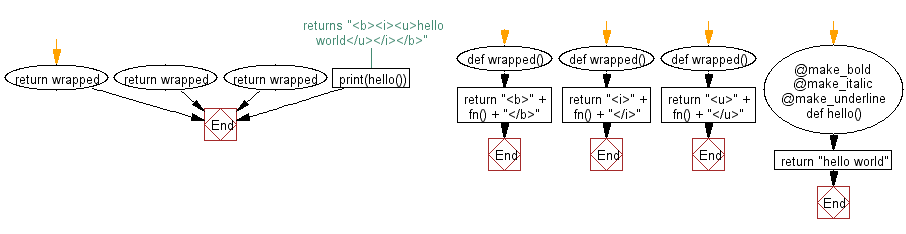﻿ Python Exercise: Make a chain of function decorators in Python - w3resource# Python Exercise: Make a chain of function decorators in Python

## Python Functions: Exercise - 17 with Solution

Write a Python program to make a chain of function decorators (bold, italic, underline etc.).

Sample Solution:-

Python Code:

``````def make_bold(fn):
def wrapped():
return "<b>" + fn() + "</b>"
return wrapped

def make_italic(fn):
def wrapped():
return "<i>" + fn() + "</i>"
return wrapped

def make_underline(fn):
def wrapped():
return "<u>" + fn() + "</u>"
return wrapped
@make_bold
@make_italic
@make_underline
def hello():
return "hello world"
print(hello()) ## returns "<b><i><u>hello world</u></i></b>"
```
```

Sample Output:

```hello world
```

Pictorial presentation:Flowchart:## Visualize Python code execution:

The following tool visualize what the computer is doing step-by-step as it executes the said program:

Python Code Editor:

Have another way to solve this solution? Contribute your code (and comments) through Disqus.

What is the difficulty level of this exercise?

Test your Programming skills with w3resource's quiz.

﻿

## Python: Tips of the Day

Decapitalizes the first letter of a string:

Example:

```def tips_decapitalize(s, upper_rest=False):
return s[:1].lower() + (s[1:].upper() if upper_rest else s[1:])
print(tips_decapitalize('PythonTips'))
print(tips_decapitalize('PythonTips', True))
```

Output:

```pythonTips
pYTHONTIPS
```